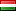Bernd07/24/2020 (Fri) 18:55:32 No.38780 del
>>38766
Wait. Let's see an example.
>Each territory has an assigned numerical value running from 1 to the number of territories.
Let's say there are 20 territories, each get a number from 1 to 20.
>In the beginning through any random process each player gets [(number of players + 1)/number of territories] numbers
Let's say there are 5 players. That means: (5+1) / 20 = 0,3?
Shouldn't that be the other way around? 20/(5+1) = 3(,33333)? So each player would get 3 territories? And the 5 which left, would become independent.